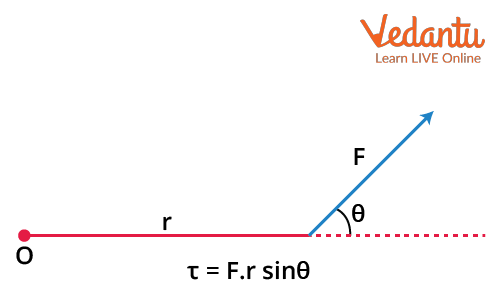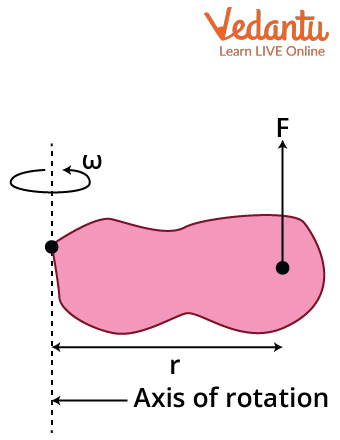Courses
Courses for Kids
Free study material
Free LIVE classes
More

# Work Done by Torque - Important Topic for JEE## What is work?

Last updated date: 28th Mar 2023
Total views: 79.5k
Views today: 1.55k

In our daily life, we often hear about work, and we even do a lot of work. Work is just something that we do that requires a bit of mental or physical effort. For example, while reading this article, you are doing some work, and this work might be beneficial for your studies. You spend energy and time reading and understanding everything that is written in this article, and you gain knowledge from it in doing so. This is what work essentially is.

In Physics, work is an important quantity. If we were to define work done, it refers to the amount of displacement that a force produces when applied to any object. Suppose you push an object by applying some force F, and it is displaced by some distance d, then the work done on the object by that force is the dot product of the force and the distance.

When you do work on any system, the energy of the system is converted from one form to another. For example, when you apply force, the kinetic energy of the object changes, and this is the work that you did on the object.

## Introduction to Torque

We all know about forces in linear motion, so torque is just the rotational analogue of the force in rotational motion. It can be called the force that causes a body to rotate about an axis. We know from Newton’s second law of motion that force causes a change in the linear acceleration of a body. Similarly, the torque causes a change in the angular acceleration of the body. The axis about which the torque makes a body rotate is known as the axis of rotation.

One could naturally ask if torque will change with the change of axis of rotation, and the answer is yes, torque changes with the axis of rotation. Torque depends on the distance between the axis of rotation and the point of application of torque, as we will see in a bit. The distance between the point of application of torque and the axis of rotation is known as the moment arm or the lever arm.Visualisation of torque

The formula for torque produced by a force F acting at a point that is at a distance r from the axis of rotation is given by the vector cross product of F and r. The mathematical form for this is

$\tau=\vec{r} \times F$

$\tau=r F \sin \theta$

The torque is represented by $\tau$ and it acts perpendicular to the plane of r and F, according to the rules of the vector product. The S.I. unit of torque is Nm.

## Work Done by Torque

First, we will write the expression for work done by a force F which displaces an object by some distance r.

$W=F \cdot r$

$W=F r \cos \theta$

This is the formula of the work done. We could be asked to give an expression for work done, and this is the most basic expression.

Suppose we have a body that is rotating with some angular velocity ω and we apply a force on it at a distance r from the axis of rotation. This force causes some angular acceleration, and the angular velocity will increase due to the torque. This will change the rotational kinetic energy of the body, so some work will be done. We can now formulate a relationship between the torque and the work done using this case.A Rotating body

We know that, according to the work-energy theorem, the amount of work done is equal to the change in kinetic energy. This theorem is also valid for rotational motion. So, the work done by all the torques will be equal to the change in kinetic energy.

$W_{\tau}=\Delta \mathrm{K} . \mathrm{E}$

Here, W𝜏 is the work done by the torque and the kinetic energy is the rotational kinetic energy. We’ll prove this equation now.

We know that work done is equal to the product of the force applied and the displacement produced by the force. Similarly, the work done by the torque can be said to be the product of the angular force applied, which is the torque, and the angular displacement produced by the applied torque. We can write this as

$W=\int \vec{\tau} \cdot d \vec{\theta}$

Work done by constant torque can be written as

$W=\tau\theta$

This is the relation between torque and work done.

We know from the section before that

$\vec{\tau}=\vec{r} \times \vec{F}$

Also, the rotational form of newton’s second law is given as

$\vec{\tau}=I \alpha$

Here, I is the rotational mass or the moment of inertia and α is the angular acceleration.

We can thus write

$W=\int I \alpha \cdot d \theta$

The angular acceleration can be written as the time derivative of angular velocity.

$\alpha=\dfrac{\mathrm{d} \omega}{\mathrm{d} t}$

And we also know that the angular velocity can be written as the time derivative of the angular displacement.

$\omega=\dfrac{\mathrm{d} \theta}{\mathrm{d} t}$

We can write the expression for work done as

\begin{align} &W=\int I \cdot \dfrac{\mathrm{d} \omega}{\mathrm{d} t} \mathrm{~d} \theta \\ &W=\int I \cdot \dfrac{\mathrm{d} \theta}{\mathrm{d} t} \mathrm{~d} \omega \\ &W=\int I \cdot \omega \mathrm{d} \omega \end{align}

If we consider $\omega_0$ as the initial angular velocity and $\omega$ as the final angular velocity, the integration gives

\begin{align} &W=I \int_{\omega_{0}}^{\omega} \omega \mathrm{d} \omega \\ &W=I\left[\dfrac{\omega^{2}}{2}\right]_{\omega_{0}}^{\omega} \\ &W=\dfrac{I \omega^{2}}{2}-\dfrac{I \omega_{0}{ }^{2}}{2} \end{align}

So, we have proved that the work done by torque is equal to the change in rotational kinetic energy of the body.

## Numerical Examples of Work Done by Torque

Example 1: A constant torque of 10 Nm produces an angular displacement of $\dfrac{\pi}{10}$ radian. Calculate the work done by this torque.

Solution: The work done by constant torque is given as

$W=\tau \theta$

Given that $\tau$=10 Nm and $\theta=\dfrac{\pi}{10}$ radian.

The work done will be

\begin{align} &W=10 \times \dfrac{\pi}{10} \\ &W=1 \mathrm{~J} \end{align}

The torque does a work of 1J.

Example 2: A wheel is rotating at an angular speed of 10 $\dfrac{rad}{s}$. It is stopped to rest by applying a constant torque for 6 seconds. If the moment of inertia of the wheel about its axis is 0.10 kg m2, then what is the magnitude of the work done by the torque.

Solution: We have been given that $\omega_0=10~\dfrac{rad}{s}$ , $\omega=0$, I=0.10 kg m2.

The work done by the torque is equal to the change in rotational kinetic energy of the body.

\begin{align} &W=\dfrac{I\omega^{2}}{2}-\dfrac{I \omega_{0}^{2}}{2} \\ &W=\dfrac{0.10}{2}\left(0-10^{2}\right) \\ &W=-\dfrac{0.10 \cdot 100}{2} \\ &W=-\dfrac{10}{2} \\ &W=-5 \mathrm{~J} \\ &|W|=5 \mathrm{~J} \end{align}

So, the torque does a work of 5J.

Example 3: A wheel rotating about an axis has a moment of inertia of 3kgm2 and a torque of 6Nm is acting on it. Find the work done by torque in t=20s starting from rest.

Solution:

We have I=3kgm2, $\tau$=6Nm.

We know that the work done by torque is given as

$W=\tau \theta$

We need to find the angular displacement in 20s.

We know that

\begin{align} &\tau=I \dfrac{\mathrm{d} \omega}{\mathrm{d} t} \\ &6=3 \times \dfrac{\mathrm{d} \omega}{\mathrm{d} t} \\ &2 d t=d \omega \end{align}

Integrating we get,

$\omega=2t$

We also know that

$\omega=\dfrac{\mathrm{d} \theta}{\mathrm{d} t}$

We need to solve this to get the angular displacement.

\begin{align} &\int_{0}^{20} \omega \mathrm{d} t=\int d \theta \\ &\int_{0}^{20} 2 t \mathrm{~d} t= \theta \\ &2\left[\dfrac{t^{2}}{2}\right]_{0}^{20}=\theta \\ &400=\theta \end{align}

The work done will be

\begin{align} &W=\tau \theta \\ &W=6 \times 800 \\ &W=4800 \mathrm{~J} \end{align}

The work done is 4800 J.

## Conclusion

Work done by a force is equal to the change in kinetic energy of the body on which the force is acting. It can also be said that the work is done to change the kinetic energy of the body. In rotational mechanics, torque is the analogue of force, and so the work done by the force should also be equal to the change in kinetic energy.

Torque is equal to the vector product of the distance of the point of application of force from the axis of rotation and the force. In the case of rotational motion, it is not the linear kinetic energy but the rotational kinetic energy of the body that is considered in the work-energy principle. So, the work done by torque is equal to the change in rotational kinetic energy of the body.

## FAQs on Work Done by Torque - Important Topic for JEE

1. Can work done be negative in Physics?

Work can either be positive or negative. If the component of force is in the same direction as the object's displacement, it is performing positive work. A force performs negative work if one of its components is in a direction that differs from the displacement. If you take up a book and gently set it back down on the floor, you will be making a negative effort because gravity is pulling the book downward, and you will be pulling it up.

2. What is the weightage of work done by torque in JEE?

Torque comes under the chapter ‘Rotational Mechanics’, and this is an extremely important topic to study from an exam point of view. The concept of torque is also used in dipoles in electrostatics, gyroscopes, etc. In rotational mechanics, torque is an integral concept, and most problems involve torque. Work done by torque is also important. Rotational mechanics has about 3.3% of the weightage in JEE, which means that almost one question is asked on this topic every year.